# 美国州内与州际能源消耗评价模型An Assessment Model of Internal and Interstate Energy Consumption of the United States

• 全文下载: PDF(1893KB)    PP.109-119   DOI: 10.12677/JLCE.2018.73013
• 下载量: 332  浏览量: 1,280   科研立项经费支持

Based on the official data of Energy Information Administration, firstly, we analyze the energy consumption of four industries from 1960 to 2009 in California, Arizona, Texas, and New Mexico from the perspective of static and dynamic, respectively. The result shows that energy consumption structure trends of the four states are basically similar. Secondly, based on the features of the states, an intrastate assessment model is proposed by using the correlation degree assessment method and TOPSIS method, which is used to measure the energy use of the states from 1960 to 2009. Finally, we propose an interstate assessment model to adapt to the development trend of the interstate alliance. Model test shows that it is necessary for the four states to sign an energy agreement for the Intercontinental Union.

1. 引言

21世纪以来，越来越多的国家将能源使用的效率视为实现经济可持续发展的重要途径。正如欧洲能源委员会所说的那样，“从长远来看，可再生能源将不可避免地在世界能源市场上占据主导地位，我们别无选择 。”自20世纪70年代初以来，许多州长都在关注增加和推广使用清洁能源和可再生能源。美国的三个能源政策法案在1992年，2005年和2007年达到顶峰 。但是，美国的能源状况因州而异，在新能源发展过程中，能源消费结构是否合理是衡量一个国家和地区经济发展水平的重要指标，也是衡量一个国家经济发展是否可持续的重要指标 。张湘宁 指出，目前美国仍处于新能源时代的过渡期，石油和天然气在能源结构中仍占有重要地位。面对国际社会日益增长的能源保护压力，美国政府还有很长的路要走。因此，能源仍是今天美国政府工作清单中的优先事项之一。能源综合分析模型在评估近期能源消费结构中越来越重要。未来的能源预测将更有利于对当前能源结构调整做出决策。董治堂 对美国的能源消费，储蓄和替代能源发现情况进行了研究。指出美国政府发展和推广替代能源是明智的行为。高静 分析了美国新能源政策的特点，认为其主要特点是发展清洁能源，减少对化石能源的依赖，改善能源结构。

2. 方法与数据来源

2.1. 关联度方法

${X}_{1}=\left\{{X}_{1}\left(1\right),{X}_{1}\left(2\right),\cdots ,{X}_{1}\left(50\right)\right\}.$ (1)

${X}_{i}=\left\{{X}_{i}\left(1\right),{X}_{i}\left(2\right),\cdots ,{X}_{i}\left(50\right)\right\},i=2,\cdots ,17.$ (2)

${{X}^{\prime }}_{i}\left(j\right)=\frac{{X}_{i}\left(j\right)}{\mathrm{max}\left\{{X}_{i}\left(1\right),{X}_{i}\left(2\right),\cdots ,{X}_{i}\left(50\right)\right\}},i=1,2,\cdots ,17;j=1,2,\cdots ,50.$ (3)

$M=\underset{i}{\mathrm{max}}\underset{k}{\mathrm{max}}|{{X}^{\prime }}_{i}\left(j\right)-{{X}^{\prime }}_{1}\left(j\right)|,\text{}i=2,3,\cdots ,17;\text{}j=1,2,\cdots ,50,$ (4)

$m=\underset{i}{\mathrm{min}}\underset{k}{\mathrm{min}}|{{X}^{\prime }}_{i}\left(j\right)-{{X}^{\prime }}_{1}\left(j\right)|,\text{}i=2,3,\cdots ,17;\text{}j=1,2,\cdots ,50.$ (5)Table 1. Explanations of second-level indexes

Tips: aIt is defined as the ratio of renewable energy total consumption to resident population (including Armed Forces) (PRECB = RETCB/TPOPP). bIt is defined as the ratio of renewable energy total consumption to total energy consumption (REURP = RETCB/TETCB). 注：a定义为可再生能源消费总量与常住人口(包括武装部队)的比率。(PRECB = RETCB/TPOPP)。b它被定义为可再生能源总消费量与总能耗之比(REURP = RETCB/TETCB)。

${r}_{i}\left(j\right)=\frac{m+\theta M}{|{{X}^{\prime }}_{1}\left(j\right)-{{X}^{\prime }}_{i}\left(j\right)|+\theta M},i=2,3,\cdots ,17,j=1,2,\cdots ,50.$ (6)

${\phi }_{1,i}=\frac{1}{50}\underset{j=1}{\overset{50}{\sum }}{r}_{i}\left(j\right),i=2,3,\cdots ,17$ (7)

$C={\left(\begin{array}{cccc}{\phi }_{1,1}& \cdots & {\phi }_{1,j}& \cdots \\ ⋮& ⋮& ⋮& ⋮\\ {\phi }_{i,1}& \cdots & {\phi }_{i,j}& \cdots \\ ⋮& ⋮& ⋮& ⋮\end{array}\right)}_{17×17}$ (8)

2.2. TOPSIS综合评价法

${Z}_{i}\left(j\right)=\frac{{X}_{i}\left(j\right)}{\sqrt{\underset{j=1}{\overset{50}{\sum }}{X}_{i}{\left(j\right)}^{2}}},j=1,2,\cdots ,50$ (9)

${Z}^{+}=\left({Z}_{1}^{+},{Z}_{2}^{+},\cdots \right)=\underset{j}{\mathrm{max}}\left\{{Z}_{i}\left(j\right)\right\},$ (10)

${Z}^{-}=\left({Z}_{1}^{-},{Z}_{2}^{-},\cdots \right)=\underset{j}{\mathrm{min}}\left\{{Z}_{i}\left(j\right)\right\}.$ (11)

${S}_{j}^{+}=\sqrt{{\sum }_{i=1}{\left({Z}_{i}\left(j\right)-{Z}_{i}^{+}\right)}^{2}},j=1,2,\cdots ,50,$ (12)

${S}_{j}^{-}=\sqrt{{\sum }_{i=1}{\left({Z}_{i}\left(j\right)-{Z}_{i}^{-}\right)}^{2}},j=1,2,\cdots ,50.$ (13)

${C}_{j}=\frac{{S}_{j}^{-}}{{S}_{j}^{-}+{S}_{j}^{+}},j=1,2,\cdots ,50.$ (14)

${C}_{j}$ 在(0,1)的范围内， ${C}_{j}$ 越大则评价对象的性能越好。在下面的过程中，我们用 ${C}_{j}$ 来评估四个研究国家在第j年的能源消费结构的表现。

2.3. 数据来源和假设

1) 能源结构只包括地球上可用的能源；

2) 自然灾害和总体政策变化不会影响50年的能源结构；

3) 人均指标相对客观；

4) 科学技术不会影响能源生产；

5) 不可再生能源储备始终大于零。

3. 能源概况分析

3.1. 能量分布静态分析

$TETCB=TECCB+TEICB+TERCB+TEACB$

$TETCB=PATCB+CLTCB+NGTCB+RETCB+NUETB$

${E}_{1}=\left\{\begin{array}{l}\text{commerce}\hfill \\ \text{transportation}\hfill \\ \text{residentialsectors}\hfill \\ \text{industrialsectors}\hfill \end{array}$ ${E}_{1}\left(\text{TETCB}\right)\left\{\begin{array}{l}\text{PATCB}\hfill \\ \text{CLTCB}\hfill \\ \text{NGTCB}\hfill \\ \text{RETCB}\hfill \\ \text{NUETB}\hfill \end{array}$

1) 加利福尼亚州工业部门能源消费总量的比例显着下降，商业部门能源消费总量的比例有所增加。而加州主要消耗石油和天然气。从20世纪70年代开始，加利福尼亚州增加了核能等清洁能源的使用比例，同时降低了石油和天然气消费的比例。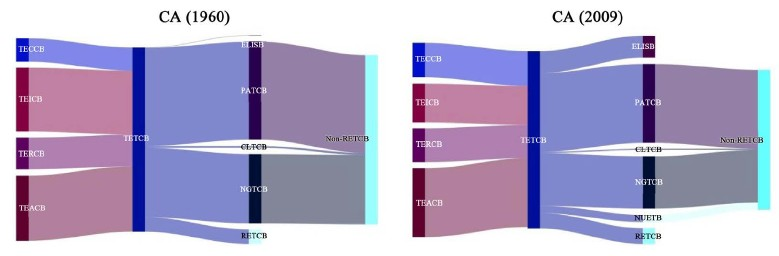Figure 1. Sankey diagram of energy diversion for California in the year 1960 (left) and 2009 (right)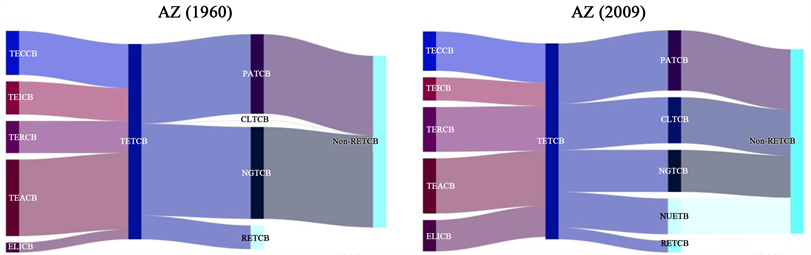Figure 2. Sankey diagram of energy diversion for Arizona in the year 1960 (left) and 2009 (right)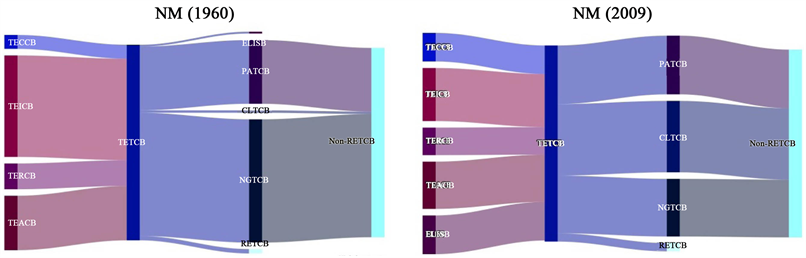Figure 3. Sankey diagram of energy diversion for New Mexico in the year 1960 (left) and 2009 (right)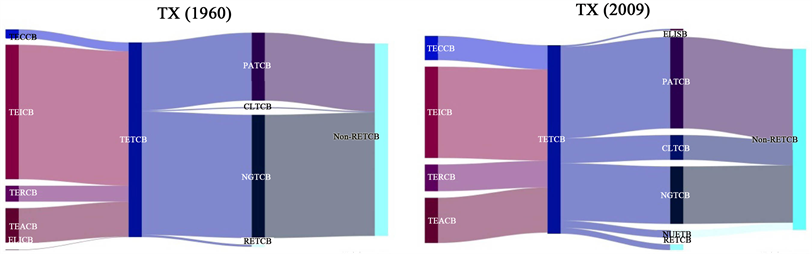Figure 4. Sankey diagram of energy diversion for Texas in the year 1960 (left) and 2009 (right)

2) 对于亚利桑那州，1960年交通运输部门的能源消耗占总数的大部分。然而，2009年各部门的能源配置更加平衡。从1960年到2009年期间，石油和天然气的比例显着下降，核能和煤炭消费的比例上升，但其石油消费仍占主导地位。

3) 对于新墨西哥州，1960年工业部门的能源消耗占总数的大部分。然而，2009年各部门的能源分配更加平衡。1960年，天然气是新墨西哥州能源消费的支柱。2009年，天然气总消耗比例下降，而煤炭总消耗比例上升。

4) 对于得克萨斯州，无论是在1960年还是在2009年，其工业部门的能源消耗仍然居各行业首位。1960年，其主要能源消费是天然气和石油。但是，2009年天然气消费比例下降。与此同时，石油消费的比例在增加，核能等清洁能源的比例也在增加。

3.2. 能量分布动态分析

1) 四个州在1960年至2009年之间都存在巨大的能源消费结构变化。具体来说，核电消耗从零开始，除新墨西哥州外，所有州都逐渐发展了核能源。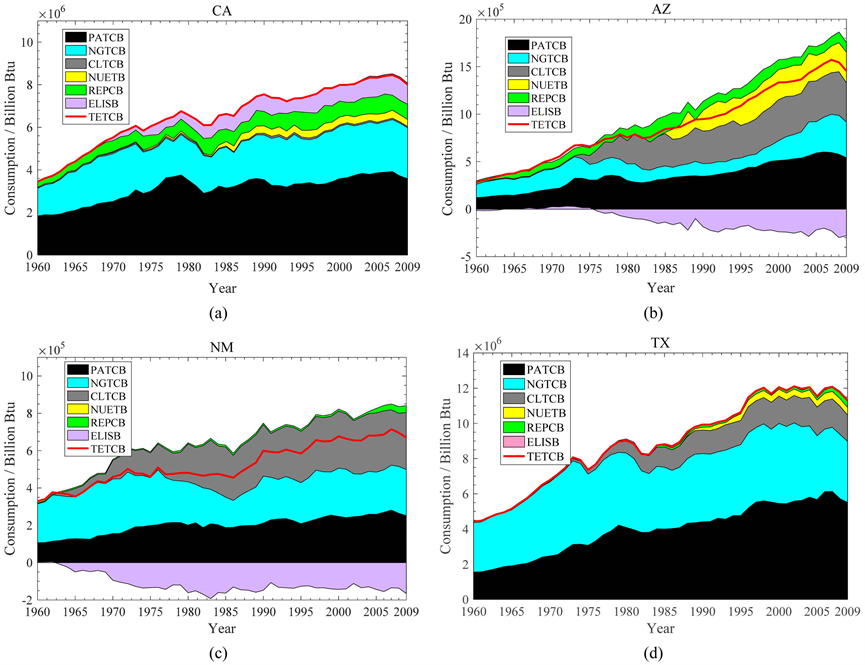Figure 5. Changes in energy consumption (TETCB) and net interstate sales of electricity and associated losses (ELISB) from the year 1960 to 2009. (a) and (d) are for California, Arizona, New Mexico, and Texas, respectively

2) 对于加州来说，1960年和2009年的州际电力和相关损失净销售额(ELISB)均为负值。然而，ELISB与总能源消费的比率(TETCB)发生了很大变化。这意味着2009年加州的电力销售面临更大的损失。亚利桑那州的可再生能源消耗量逐渐增加(见图5(b))，但由于可再生能源消费量占能源消费总量的比例大幅下降，不可再生能源消费量大幅度增加。

3) 新墨西哥州的ELISB在50年内(从1960年到2009年)从正值变为负值，而德克萨斯州的情况正好相反。2009年的ELISB数据显示，新墨西哥州在50年后获得更多的电力销售盈余。

4) 四个州的总能量消耗逐渐增加(图5中的红色实线)，但不单调。包括石油PATCB，NGTCB，CLTCB和NUETB的不可再生能源消耗在这四个州的总能耗中占据主导地位，尤其是新墨西哥州和德克萨斯州。

5) 相比之下，加州和亚利桑那州的清洁能源消耗大幅增加，如REPCB和NUETB，这意味着这两个州的能源结构已经发生了变化。然而，其他两个州的清洁能源消耗很少。亚利桑那州和新墨西哥州的ELISB均为负值，这表明这两个州的电力销售有盈余。

4. 能源评价模型

4.1. 州内评价模型的结果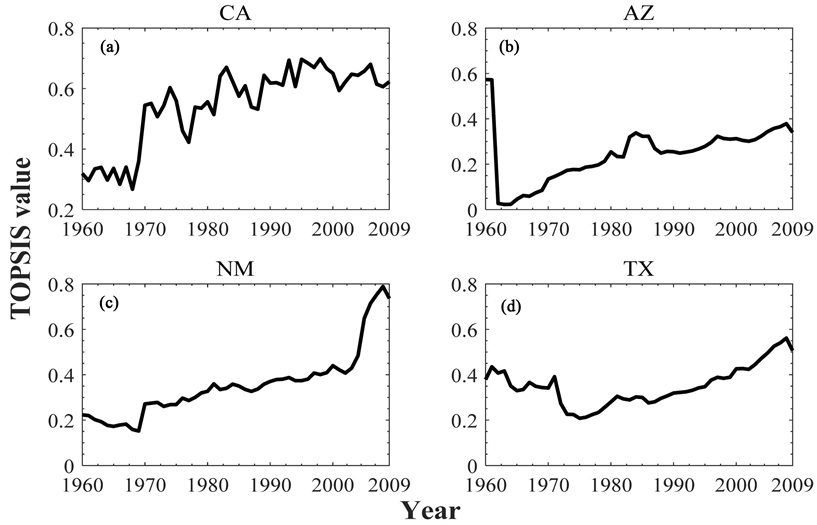Figure 6. TOPSIS values of four states in the years 1960~2009. (a)~(d) denote California, Arizona, New Mexico, and Texas by using individualized indexes, respectivelyTable 2. Selected most influential indexes for the four studied states

1) 对于加利福尼亚州来说，60年代TOPSIS值相对较低。然而，1970年出现上涨，此后该值接近较高水平，这是因为政府逐渐重视清洁能源的使用。同时，石油工业在能源消费中占据主要地位，这可能抵消了可再生能源的优势，导致了1970~2009年间的剧烈波动。一个有趣的发现是，由于电力销售的巨大损失，TOPSIS值在2000~2001年间骤降，这与加州2000年6月爆发了二战后美国最大的电力危机这一事实相吻合 。

2) 亚利桑那州1960年的TOPSIS值非常高，但从1961年起在出现了暴跌。这是因为1960年煤炭消耗量很少(约200亿BTU)，但在1961年其消耗总量相对于1960年已经扩大了30倍。因此，这种不可再生能源消耗的增加损害了能源结构，降低了TOPSIS值。

3) 新墨西哥州有一个相对稳定的时期(1960~2000年)。这表明41年来该州能源利用效率稳定。然而，这一时期的TOPSIS值相对较低，这是由于能源开发高度依赖于石油产品消费和煤炭消费。然而，过去10年TOPSIS价值的大幅放大，受益于能源消费结构的调整，即可再生能源在能源消费总量中的比重逐渐增加。

4) 20世纪70年代，得克萨斯州的TOPSIS值出现了下滑，这是由于煤炭的过度消耗造成的。然而，从1978年开始，通过减少煤炭能源消耗，能源消费结构得到改善。

4.2. 州际评价模型的结果

4.3. 模型检验

5. 结语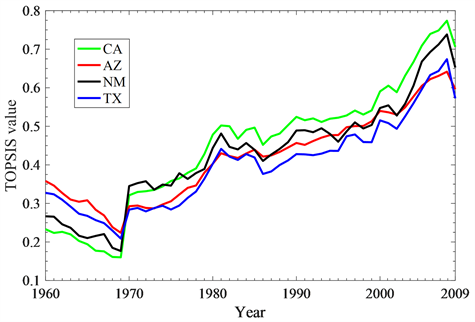Figure 7. TOPSIS value of four states calculated by using four public index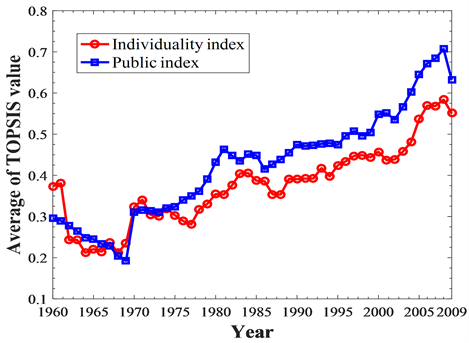Figure 8. Average of TOPSIS value calculated by the individuality index and public index

NOTES

*通讯作者。

  Earthscan (2010) Renewable Energy in Europe: Markets, Trends, and Technologies. Encyclopedia of Energy, 9, 313-331.  于文轩. 美国能源安全立法及其对我国的借鉴意义[J]. 中国政法大学学报, 2011(6): 119-129.  侯佳儒. 美国可再生能源立法及其启示[J]. 郑州大学学报(哲学社会科学版), 2009, 42(6): 79-84.  张湘宁. 美国能源新政对我们的启示[J]. 西南石油大学学报(社会科学版), 2009, 2(1): 15-20.  董治堂. 中美能源政策对比研究[J]. 经济经纬, 2007(1): 61-65.  高静. 美国新能源政策分析及我国的应对策略[J]. 世界经济与政治论坛, 2009(6): 58-61.  郝新东. 中美能源消费结构问题研究[D]: [博士学位论文]. 武汉: 武汉大学, 2013.  李彦斌, 于心怡, 王致杰. 采用灰色关联度与TOPSIS法的光伏发电项目风险评价研究[J]. 电网技术, 2013, 37(6): 1514-1519.  李荣平. 基于灰色关联度方法的科技进步效果评价与分析——以河北省为例[J]. 改革与战略, 2007, 23(10): 33-36.  邹晓雯. 水质评价的灰色关联度方法[J]. 水资源保护, 1994(3): 11-16.  Doukas, H., Karakosta, C. and Psarras, J. (2009) A Linguistic TOPSIS Model to Evaluate the Sustainability of Renewable Energy Options. International Journal of Global Energy Issues, 32, 102-118. https://doi.org/10.1504/IJGEI.2009.027976  方鹏骞, 张治国, 杨梅. TOPSIS法在医院绩效评价中的应用[J]. 中国卫生统计, 2005, 22(3): 169-170.  胡永宏. 对TOPSIS法用于综合评价的改进[J]. 数学的实践与认识, 2002, 32(4): 572-575.  U.S. Energy Information Administration (2016) Appendix A. Mnemonic Series Names (MSN). https://www.eia.gov/state/seds/sep_use/notes/use_a.pdf  Wang, F. (2016) A Novel Coefficient for Detecting and Quantifying Asymmetry of California Electricity Market Based on Asymmetric Detrended Cross-Correlation Analysis. Chaos: An Interdisciplinary Journal of Nonlinear Science, 26, 280-286. https://doi.org/10.1063/1.4953012  Christensen, J. (2015) Book Review: Rome, The Genius of Earth Day: How a 1970 Teach-In Unexpectedly Made the First Green Generation, by Jon Christensen. Pacific Historical Review, 84, 368-369. https://doi.org/10.1525/phr.2015.84.3.368  邵志刚. 美国石油政策的演变与战略石油储备政策的形成初探[D]: [硕士学位论文]. 苏州: 苏州大学, 2008.  汪利娜. 美国次级抵押贷款危机的警示[J]. 中国房地产金融, 2007(10): 1-8.